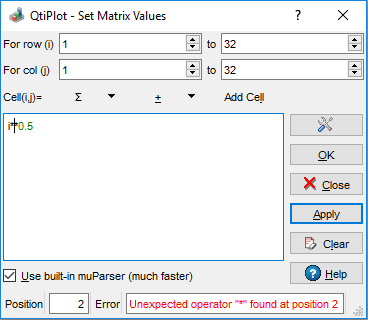# Set Matrix Values

This command is located in the Matrix menu. It allows filling in a matrix with the results of evaluating a function, z=f(i,j), in which i and j are the row and column numbers.

You can use the X-values and Y-values defined with the Set Dimensions... command, and also define your functions based on the x and y variables.

Functions can span several lines. The available intrinsic functions are listed in the muParser section of the Mathematical Expressions and Scripting chapter.

Figure 5-173. The Set Values... dialog for matrix.Using Python as scripting engine for the calculation of matrix values has the advantage of a more powerful syntax. The current matrix can be accessed using the self key word, like in the script example bellow, which fills the matrix with random values:

```from random import random
for i in range (1, self.numRows() + 1):
for j in range (1, self.numCols() + 1):
self.setCell(i, j, random())
```

For more details on the Python API available in QtiPlot, see the section on Python in the chapter on Mathematical Expressions and Scripting . The drawback of using Python as scripting engine is that it can be quite slow for large matrices. You can use muParser instead, even if Python is set as the default script engine for your QtiPlot project, by checking the Use built-in muParser box. Note that muParser is very fast for the evaluation of single line expressions only, therefore try to avoid syntax like:

```	a = cell(1, 1)
b = cell(2, 2)
a*b*x + b*x*x + a
```

in preference for something like the following:

```	cell(1, 1)*cell(2, 2)*x + cell(2, 2)*x*x + cell(1, 1)
```

which will greatly increase the speed of evaluation!

The status bar at the bottom of this dialog displays the current Position of the editing cursor as well as any error messages that might occur during the evaluation of the user provided function.